# 14. Matching.In the overlapping region we letand introduce the intermediate variable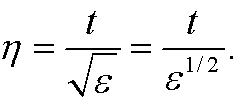This is a time scale that is “between” de innerand outer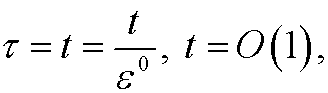time scales. To be able to match the approximations we require that the outer and inner approximations (written with respect to the intermediate variable) must agree in the limitthat isfor a fix (positive) value of. in our case this means thatVWe realize that a=e and that our inner approximation therefore must be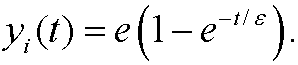Finally, we want to find a solution that is valid in all the interval [0,1]. We therefore construct yu from the inner and outer approximations minus their common limit e (since it otherwise would have been counted twice) in the overlapping region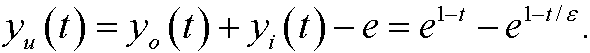When t is in the outer region the second term is small and yu is thus approximatelywhich is exactly the outer approximation. When t is in the boundary layer the first term is close to e and yu is thus approximately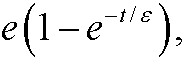which is exactly the inner approximation. In the overlapping region both the inner and outer approximations are approximately equal to e, which makes the sum of  yi and yo close to 2e there, that is, twice as much as it should be. That is why we have to subtract the common limit from the sum. If we insert yu in the original differental equation we see thatdthat is, yu fulfills the equation exactly on the interval (0,1). If we investigate the boundary conditions we see thatThe left condition is exactly fulfilled and the right is fulfilled up to´for any n>0 , sinceWe thus see that yu is a unitly good approximation on [0,1].